Intermediate Geometry : Tangent Lines

Example Questions

2 Next →

Example Question #1 : How To Find The Slope Of A Tangent Line

Suppose the equation of a line is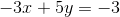.  What is the slope of the tangent line at?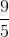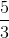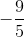Explanation:

Rewritein slope-intercept form,.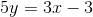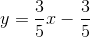The slope of the tangent line is.

Example Question #2 : How To Find The Slope Of A Tangent Line

Suppose a function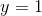.  What is the slope of the tangent line at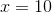?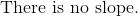Explanation:

Write the formula for slope-intercept form.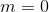The slope ofis always zero at every point on the domain.  Therefore, the slope atmust also be zero.

2 Next →